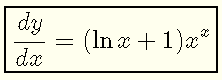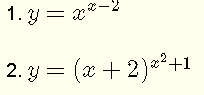# Find The Derivative of x x

Find the first derivative of y = x x for x > 0 with all the steps presented.

## Derivative of x x with Steps

Note that the function y = x x is neither a power function of the form x k nor an exponential function of the form b x and the known formulas of Differentiation of these two functions cannot be used. We need to find another method to find the first derivative of the given function.
GivenTake the natural log (ln) of both sides of the aboveUse properties of logarithmic functions
ln Ab = b ln A   to the right side of the above equation and obtainDifferentiate both sides of the above with respect to
x , using the chain rule on the left side and the product rule on the right.Simplify the right sideMultiply both sides by
y and simplifySubstitute
y by x x to obtain the final answer## Exercises

Find the first derivative of